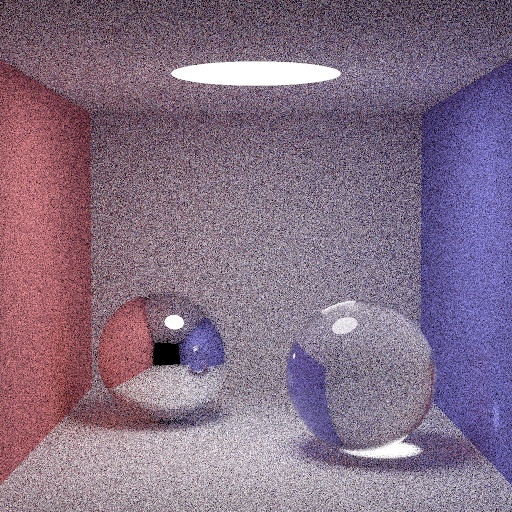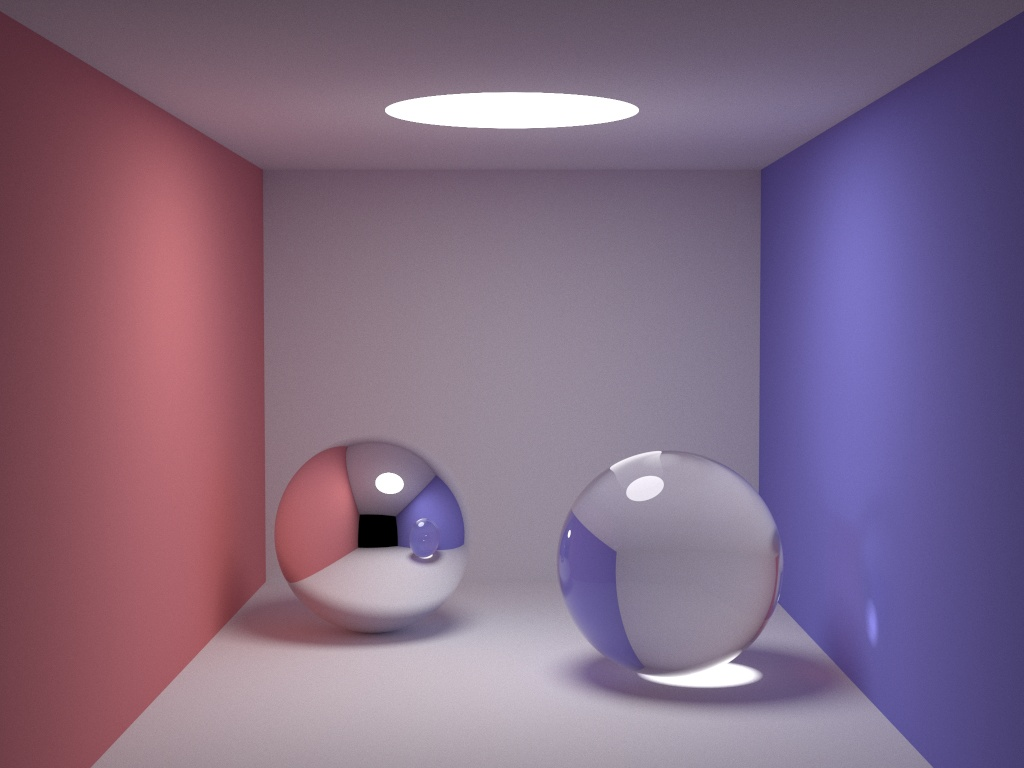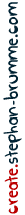Interactive demos require Javascript. Anything else works.

# smallpt - Global Illumination in Javascript

posted by Stephan Brumme

## smallpt

Kevin Beason published a C++ Monte Carlo path tracer that is just 99 lines long short. Despite this limit the code supports the most common materials: diffuse and specular reflection as well as refraction.
Sven Klose, apparently from Germany like me, ported the code to Javascript but his website is down for quite some time now. I couldn't find his source code anywhere on the internet so I converted the code from C++ to Javascript again and compared how fast it runs on the most important web browsers.

## Live Demo

You need a web browser with HTML5-canvas support. It's a standard feature of all modern browsers and you are typically fine even with slightly older versions of Chrome, Opera, Safari or Firefox. However, Internet Explorer needs to be version 9+ which is not available for the still popular Windows XP.

passes:

original size (64x64):

8x zoomed (512x512):
Oops, your browser doesn't support HTML5 canvas ...

## Benchmark

Path tracers never finish their computations. If you are looking for the perfect image then you need to wait until Last Judgment.
I arbitrarily chose to abort after each pixel was oversampled 100 times.

The web browsers' Javascript engines exhibit huge performance differences:
100 passes version seconds samples/second
Firefox 7 18.9 21.6k
10 4.4 87.1k
Opera 11.60 9.9 41.4k
Internet Explorer 9 17.8 23.0k
Update November 28, 2011: The brandnew Firefox 9 is four times faster than Firefox 8.

My computer is equipped with a Intel Core i7 860 @ 2.8GHz. All browsers use only 1 (of 8 available) cores. Even though my Windows 7 installation is 64-bit, all browsers are still 32-bit.

Overhead for displaying the original 64x64 pixel rendering and the zoomed 512x512 image is about 1 second for each browser, only Opera is a bit slower at 1.5 seconds. The numbers in the table include this overhead to allow for better comparison with your own results.

Frankly, I'm quite disappointed by Firefox. Chrome is currently the fastest browser by far for this benchmark and Opera is doing surprisingly well. From a usuability perspective, Chrome is the only browser where I didn't notice a severe lagging of mouse movements while the rendering is still in progress.

## Original C++ Version

The C++ version needs about 21 seconds to generate a 512x512 (instead of 64x64) image with 100x oversampling. That's equivalent to 1.2 million samples per second or roughly 10x faster than Chrome. It's only fair to add that the C++ version spreads the work to all available cores thanks to OpenMP. When disabling OpenMP, the C++ version needs 76 seconds (344k samples/seconds), 3x faster than Chrome.

Below is the resulting image (converted to progressive JPEG, quality set to 95% to save bandwidth):After a few hours pretty much all noise is gone (1024x768, 5000 samples/pixel, image courtesy of Kevin Beason):## Source Code

The original C++ source code is publicly available on Kevin's homepage. It can compile to a (Linux) binary weighting less than 4k.
Unlike my Javascript port it does not display the rendered image but writes it straight to disk in the PPM file format. Irfanview and XnView are popular choices to display PPMs.

I did not a 1:1 port because I soon figured out I'll never fit the Javascript version into 99 lines (without sacrificing readability). Instead I chose longer, more descriptive names for variables, added comments and quite some whitespace. The whole `radiance` function was split into diffuse, specular and refractive sub-routines.
The HTML5 canvas overhead is about 60 lines, that means the core code is three times bigger in Javascript. If you really want to, you can certainly squeeze everything in 99 lines as well, but I doubt it will look nice ...

``` "use strict"; // original C++ version: smallpt, a Path Tracer by Kevin Beason, 2008 // this Javascript port: Stephan Brumme, 2011 // two drawing areas: one is enlarged (canvas2), one is at original size (canvas) var canvas = document.getElementById('myCanvas'); var canvas2 = document.getElementById('myCanvasZoomed'); var stretchX = canvas2.width / canvas.width; var stretchY = canvas2.height / canvas.height; var width = canvas.width; var height = canvas.height; var context = canvas .getContext('2d'); var context2 = canvas2.getContext('2d'); context .fillText('(Press Start)', canvas .width/2-28, canvas .height/2); context2.fillText('(Press "Start Rendering !")', canvas2.width/2-50, canvas2.height/2); context2.scale(stretchX, stretchY); // get direct access to pixels var imageData = context.getImageData(0, 0, canvas.width, canvas.height); var image = imageData.data; // show some info on canvas2 context2.font = '10px sans-serif'; context2.textAlign = 'center'; context2.fillStyle = '#99FF99'; var maxSamples = 100; // passes var epsilon = 0.0001; // accuracy var startTime = 0; var inProgress = false; var stop = false; // RGB color of a single pixel function Color(r, g, b) { this.r = r; this.g = g; this.b = b; } Color.prototype.toString = function() { return 'rgb('+this.r+','+this.g+','+this.b+')'; } Color.prototype.scaled = function(s) { return new Color(this.r*s, this.g*s, this.b*s ); } Color.prototype.plus = function(c) { return new Color(this.r+c.r, this.g+c.g, this.b+c.b); } Color.prototype.minus = function(c) { return new Color(this.r-c.r, this.g-c.g, this.b-c.b); } Color.prototype.filtered = function(c) { return new Color(this.r*c.r, this.g*c.g, this.b*c.b ); } Color.prototype.clamp = function(s) { if (s < 0) return 0; if (s > 1) return 1; return s; } Color.prototype.clamped = function() { return new Color(this.clamp(this.r), this.clamp(this.g), this.clamp(this.b)); } Color.prototype.gamma = function(s) { var inv = 1/s; return new Color(Math.pow(this.r, inv), Math.pow(this.g, inv), Math.pow(this.b, inv)); } // 3D vector function Vector(x, y, z) { this.x = x; this.y = y; this.z = z; } Vector.prototype.length = function() { return Math.sqrt (this.x*this.x + this.y*this.y + this.z*this.z); } Vector.prototype.toString = function() { return 'v('+this.x+','+this.y+','+this.z+')'; } Vector.prototype.reverse = function() { return new Vector(-this.x, -this.y, -this.z); } Vector.prototype.scaled = function(s) { return new Vector(this.x*s, this.y*s, this.z*s ); } Vector.prototype.plus = function(v) { return new Vector(this.x+v.x, this.y+v.y, this.z+v.z); } Vector.prototype.minus = function(v) { return new Vector(this.x-v.x, this.y-v.y, this.z-v.z); } Vector.prototype.cross = function(v) { return new Vector(this.y*v.z - this.z*v.y, this.z*v.x-this.x*v.z, this.x*v.y - this.y*v.x); } Vector.prototype.dot = function(v) { return this.x*v.x + this.y*v.y + this.z*v.z ; } Vector.prototype.normalized = function() { return this.scaled(1 / this.length()); } // some often used constants var Black = new Color (0,0,0); var CoordX = new Vector(1,0,0); var CoordY = new Vector(0,1,0); // ray class, must have origin and direction, can have distance function Ray(origin, direction) { this.origin = origin; this.direction = direction; this.distance = 0; } Ray.prototype.hitPoint = function() { return this.origin.plus(this.direction.scaled(this.distance)); } // sphere class function Sphere(radius, center, color, emission, reflection) { if (emission == null) emission = Black; this.radius = radius; this.center = center; this.color = color; this.emission = emission; this.reflection = reflection; } Sphere.prototype.normal = function(hitPoint) { return hitPoint.minus(this.center).normalized(); } Sphere.prototype.intersect = function(ray) { // returns distance, 0 if no hit // by solving t^2*d.d + 2*t*(o-p).d + (o-p).(o-p)-R^2 = 0 var op = this.center.minus(ray.origin); var b = op.dot(ray.direction); var det = b*b - op.dot(op) + this.radius*this.radius; if (det < 0) return 0; // sphere/ray-intersection gives two solutions var t; det = Math.sqrt(det); t = b - det; if (t > epsilon) return t; t = b + det; if (t > epsilon) return t; // no hit return 0; } Sphere.prototype.diffuse = function(ray, depth) { // ray/sphere intersection var hitPoint = ray.hitPoint(); var normal = this.normal(hitPoint); var forward = normal.dot(ray.direction) < 0 ? normal : normal.reverse(); // generate new random ray var r1 = 2*Math.PI*Math.random(); var r2 = Math.random(); var r2s = Math.sqrt(r2); var w = forward; var u = (Math.abs(w.x)>0.3 ? CoordX : CoordY).cross(w).normalized(); var v = w.cross(u); var d = u.scaled(Math.cos (r1)*r2s) .plus( v.scaled(Math.sin (r1)*r2s)).plus( w.scaled(Math.sqrt(1-r2))).normalized(); return this.emission.plus(this.color.filtered(radiance(new Ray(hitPoint, d), ++depth))); } Sphere.prototype.specular = function(ray, depth) { // ray/sphere intersection var hitPoint = ray.hitPoint(); var normal = this.normal(hitPoint); var reflected = new Ray(hitPoint, ray.direction.minus(normal.scaled(2*normal.dot(ray.direction)))); return this.emission.plus(this.color.filtered(radiance(reflected, ++depth))); } Sphere.prototype.refraction = function(ray, depth) { // ray/sphere intersection var hitPoint = ray.hitPoint(); var normal = this.normal(hitPoint); var forward = normal.dot(ray.direction) < 0 ? normal : normal.reverse(); var reflected = new Ray(hitPoint, ray.direction.minus(normal.scaled(2*normal.dot(ray.direction)))); var entering = normal.dot(forward) > 0; // ray from outside going in ? var air = 1; var glass = 1.5; var refraction = entering ? air/glass : glass/air; var angle = ray.direction.dot(forward); var cos2t = 1 - refraction*refraction*(1-angle*angle); if (cos2t < 0) // total internal reflection return this.emission.plus(this.color.filtered(radiance(reflected, depth))); var tdir = ray.direction.scaled(refraction).minus( normal.scaled((entering?+1:-1)*(angle*refraction+Math.sqrt(cos2t)))).normalized(); var a = glass - air; var b = glass + air; var c = 1 - (entering ? -angle : tdir.dot(normal)); var R0 = a*a/(b*b); var Re = R0 + (1-R0)*c*c*c*c*c; var Tr = 1 - Re; var P = .25 + 0.5*Re; var RP = Re / P; var TP = Tr / (1-P); depth++; if (depth <= 2) return this.emission.plus(this.color.filtered(radiance( reflected,depth).scaled(Re).plus(radiance( new Ray(hitPoint, tdir), depth).scaled(Tr)))); if (Math.random() < P) return this.emission.plus(this.color.filtered(radiance( reflected ,depth).scaled(RP))); else return this.emission.plus(this.color.filtered(radiance( new Ray(hitPoint, tdir), depth).scaled(TP))); } // find nearest intersection by processing all spheres function intersect(ray) { var sphere = null; var minDistance = 1e10; // infinity for (var i = 0; i < spheres.length; i++) { var distance = spheres[i].intersect(ray); // no self-intersection and closer than previous hit ? if (distance > epsilon && distance < minDistance) { minDistance = distance; sphere = spheres[i]; } } // no intersection ? if (sphere == null) return null; // adjust ray and return hit object ray.distance = minDistance; return sphere; } // get radiance after ray was adjusted by intersection function radiance(ray, depth) { // find closest sphere intersecting the ray var sphere = intersect(ray); if (sphere == null) return Black; if (depth > 20) return sphere.color; if (depth > 2) { // max. reflective color channel var maxReflection = Math.max(sphere.color.x, sphere.color.y, sphere.color.z); if (Math.random() < maxReflection) { var result = sphere.color; result = result.scaled(1/maxReflection); } else return sphere.color; } return sphere.reflection(ray, depth); } // build scene var spheres = new Array( new Sphere( 1e5, new Vector(+1e5+1, 40.8, 81.6), new Color(.75,.25,.25), null, Sphere.prototype.diffuse), // left new Sphere( 1e5, new Vector(-1e5+99, 40.8, 81.6), new Color(.25,.25,.75), null, Sphere.prototype.diffuse), // right new Sphere( 1e5, new Vector(50, 40.8, 1e5 ), new Color(.75,.75,.75), null, Sphere.prototype.diffuse), // back new Sphere( 1e5, new Vector(50, 40.8,-1e5+170), Black, null, Sphere.prototype.diffuse), // front new Sphere( 1e5, new Vector(50, 1e5, 81.6), new Color(.75,.75,.75), null, Sphere.prototype.diffuse), // top new Sphere( 1e5, new Vector(50, -1e5+81.6, 81.6), new Color(.75,.75,.75), null, Sphere.prototype.diffuse), // bottom new Sphere(16.5, new Vector(27, 16.5, 47 ), new Color(.999,.999,.999), null, Sphere.prototype.specular), // mirror new Sphere(16.5, new Vector(73, 16.5, 78 ), new Color(.999,.999,.999), null, Sphere.prototype.refraction), // glass new Sphere(600, new Vector(50, 681.33, 81.6), Black, new Color(12,12,12), Sphere.prototype.diffuse) // light ); // camera var camera = new Ray(new Vector(50, 52, 295.6), new Vector(0, -0.042612, -1).normalized()); var cx = new Vector(width*0.5135/height, 0, 0); var cy = cx.cross(camera.direction).normalized().scaled(-0.5135); // compute one pass var pass; var accumulate; function compute() { var weight = 1/pass; for (var y = 0; y < height; y++) for (var x = 0; x < width; x++) { // initial ray, based on camera var d = cx.scaled(x/width - 0.5).plus(cy.scaled(y/height - 0.5)); d = d.plus(camera.direction).normalized(); // start tracing the ray var color = radiance(new Ray(camera.origin.plus(d.scaled(140)), d), 0); color = color.clamped(); // add all results (and later divide by number of passes) for averaging / noise elimination var offset = y*width+x; accumulate[offset] = accumulate[offset].plus(color); // set R, G, B values var smooth = accumulate[offset].scaled(weight).gamma(2.2); offset *= 4; image[offset++] = 255.99*smooth.r; image[offset++] = 255.99*smooth.g; image[offset ] = 255.99*smooth.b; } // blit image context.putImageData(imageData, 0, 0); context2.drawImage(canvas, 0, 0); // show some statistics var duration = (new Date().getTime() - startTime) / 1000; var expected = duration*(maxSamples)/(pass-1+y/height+0.0001); var throughput = (y+(pass-1)*height)*width/duration; context2.scale(1/stretchX, 1/stretchY); context2.fillText(Math.round(duration)+'s (of '+Math.round(expected)+'s), pass '+pass+', '+ Math.round(throughput)+' samples/s', canvas2.width/2, canvas2.height-5); context2.scale(stretchX, stretchY); // next pass, call myself via zero-timer if (pass < maxSamples && !stop) { pass++; // if calling compute() directly then screen wouldn't be updated setTimeout('compute();', 0); } else { // rendering finished, show final statistics context.putImageData(imageData, 0, 0); context2.drawImage(canvas, 0, 0); context2.scale(1/stretchX, 1/stretchY); context2.fillText('done: '+duration+'s, '+pass+' samples/pixel', canvas2.width/2, canvas2.height-5); context2.scale(stretchX, stretchY); document.getElementById('startStop').value = 'Start Rendering !'; stop = false; inProgress = false; } } // start rendering function smallpt() { // signal stop if (inProgress) { stop = true; return; } // user-defined number of passes var userPasses = document.getElementById('numPasses').value; if (userPasses > 0) maxSamples = userPasses; else { alert("Invalid number of passes !"); return; } startTime = new Date().getTime(); // wall clock inProgress = true; stop = false; // initialize color buffers accumulate = new Array(); for (var i = 0; i < width*height; i++) accumulate[i] = Black; // take care of Alpha channel: 255 => fully opaque for (var i = 0; i < width*height; i++) image[4*i+3] = 255; // start first pass pass = 1; document.getElementById('startStop').value = 'Stop Rendering !'; compute(); } ```